# [GSoC2018|OrbitDeterminator|Jorge] Angles-only orbit determination: Gauss method and least-squares

## 1. Introduction

During GSoC 2018, I worked on developing a ra/dec angles-only Keplerian orbit determination implementation for AerospaceResearch.net’s orbitdeterminator project. Our implementation incorporates both elements from the Gauss method for orbit determination, as well as from the least-squares principle. I did this both for Earth-orbiting bodies (satellites, space debris, etc.), and Sun-orbiting bodies (asteroids, comets, centaurs, etc.).

My implementation makes use of the `astropy` library, whose `SkyCoord` class provided the adequate abstraction in order to perform the required computations. `astropy` was also really useful for handling of units, angles, longitudes, etc., as well as providing access to the SPK kernel of JPL ephemerides DE432s, which are at the core of the orbit determination for Sun-orbiting bodies. Also, the `poliastro` library was useful for the evaluation of Stumpff’s functions in order to perform the refinement stages of the Gauss method. Finally, `scipy`’s functionality allowed the use of the Levenberg-Marquardt method for the least-squares fit to ra/dec observations.

## 2. Link to my GSoC 2018 project work:

The main part of the work I did is contained in the following modules:

• `orbitdeterminator/kep_determination/gauss_method.py`
• `orbitdeterminator/kep_determination/least_squares.py`

The pull request associated to my GSoC project is in GitHub at: aerospaceresearch/orbitdeterminator PR #141. My GitHub handle is @PerezHz . You can also find me on Twitter as @Perez_Hz.

## 3. Gauss method

The `orbitdeterminator/kep_determination/gauss_method.py` module allows the user to input files which contain ra/dec observations of either Earth-orbiting or Sun-orbiting celestial bodies, following specific formats for each case, and allows to compute an orbit from `n>=3` observations.

If the number of observations is `n==3`, then the Gauss method is applied to these 3 observations. Otherwise, if `n>3`, then the Gauss method is executed over `n-2` consecutive observation triplets. A set of orbital elements is computed for each of these triplets, and an average over the resulting `n-2` set of elements is returned. A refinement stage has been implemented for the Gauss method such at each iteration of the refinement stage, a better approximation for Lagrange’s f and g functions are computed; which in turn allows to get better estimates for the cartesian state of the observed body.

The right ascension and declination observations topocentric, i.e., they are referred to registered sites either by the MPC or COSPAR. The information about the geocentric position of these sites is contained in registered lists. Both the IOD and MPC formats contain the alphanumeric codes associated to each observation site, so that for each observation it is possible to compute the geocentric position of the observer. This whole process is automatically processed during runtime in the background, so the user does not need to worry about these details.

### 3.1 Earth-orbiting bodies: `gauss_method_sat`

The `gauss_method_sat` function computes Keplerian elements from ra/dec observations of an Earth-orbiting body. The call signature is the following:

`gauss_method_mpc(filename, bodyname, obs_arr, r2_root_ind_vec=None, refiters=0, plot=True)`

Where `filename` refers to the name of the file which contains the observations in IOD-format, `bodyname` is an user-defined name for the observed object, `obs_arr` is a list/array of integers which define the numbers of the lines in the observation file `filename` which are going to be processed, `r2_root_ind_vec` allows the user to select the roots whenever multiple solutions to the Gauss polynomial exist (default behavior is to select always the first root), `refiters` is the number of iterations for the refinement stage of the Gauss method (default value is `0`), and `plot` is a flag which if set to `True` will plot the orbit of the object around the Earth. More details may be found at the examples and the documentation. The orbital elements are returned, respectively, in kilometers, UTC seconds and degrees, and they are referred to the (equatorial) ICRF frame. More details may be found at the examples and the documentation.

Example output: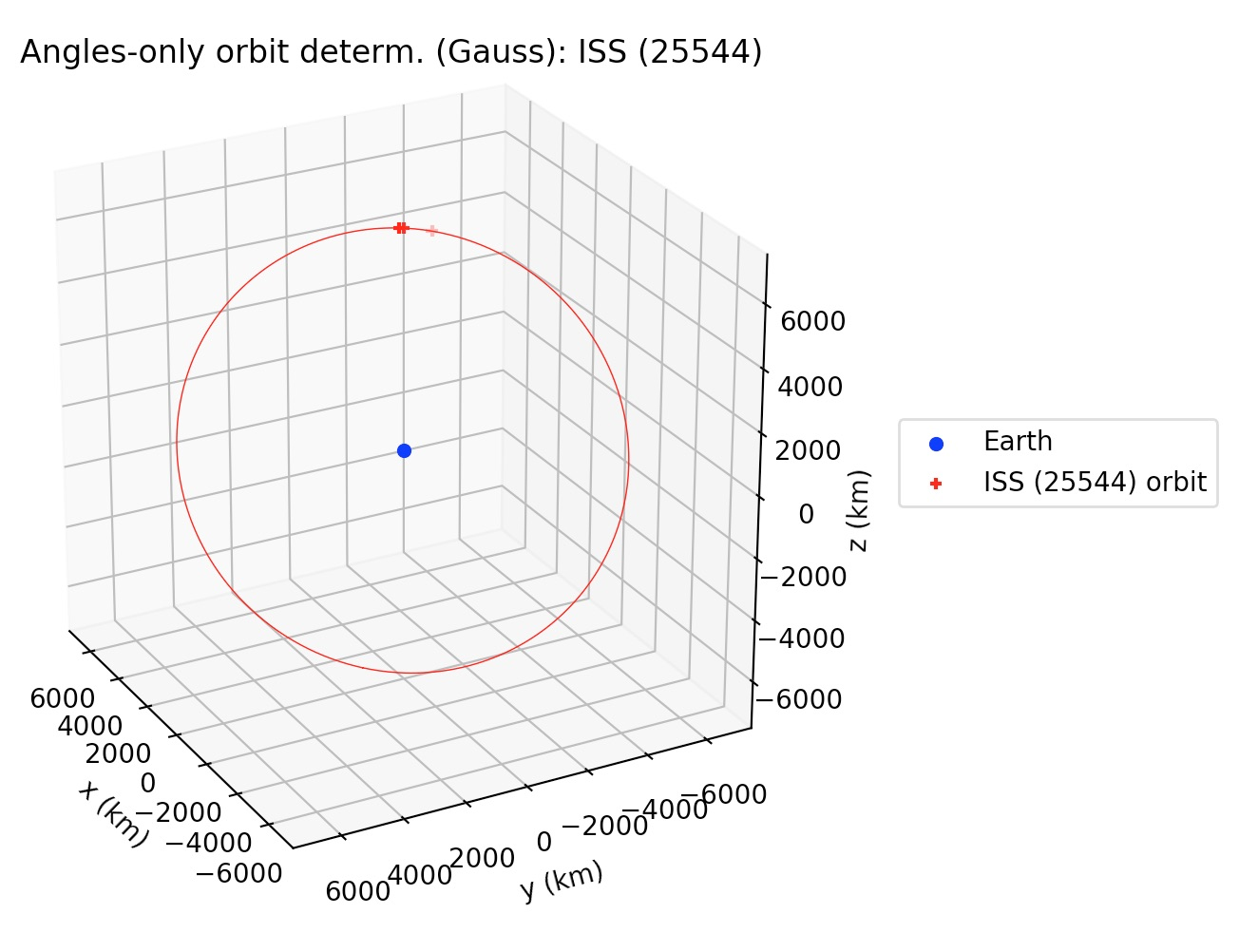### 3.2 Sun-orbiting bodies: `gauss_method_mpc`

The `gauss_method_mpc` function computes Keplerian elements from ra/dec observations of an Earth-orbiting body. The call signature is practically same as `gauss_method_sat` (see section 3.1). One of the differences with respect to the Earth-orbiting case is that in this case the orbital elements are returned, respectively, in astronomical units, TDB days and degrees. But the main difference in the internal processing is that in this case, since the orbit is referred to the Sun, then the position of the Earth with respect to the Sun has to be determined at each of the observation times. `gauss_method_mpc` uses the JPL DE432s, as provided by the `astropy` library, in order to compute the heliocentric position of the Earth. This also involves a conversion from the UTC to the TDB time scale, which is also handled again using `astropy`’s time scale conversion functionalities. Finally, a third difference is that Sun-orbiting orbital elements are returned in the mean J2000.0 ecliptic heliocentric frame. More details may be found at the examples and the documentation.

Example output: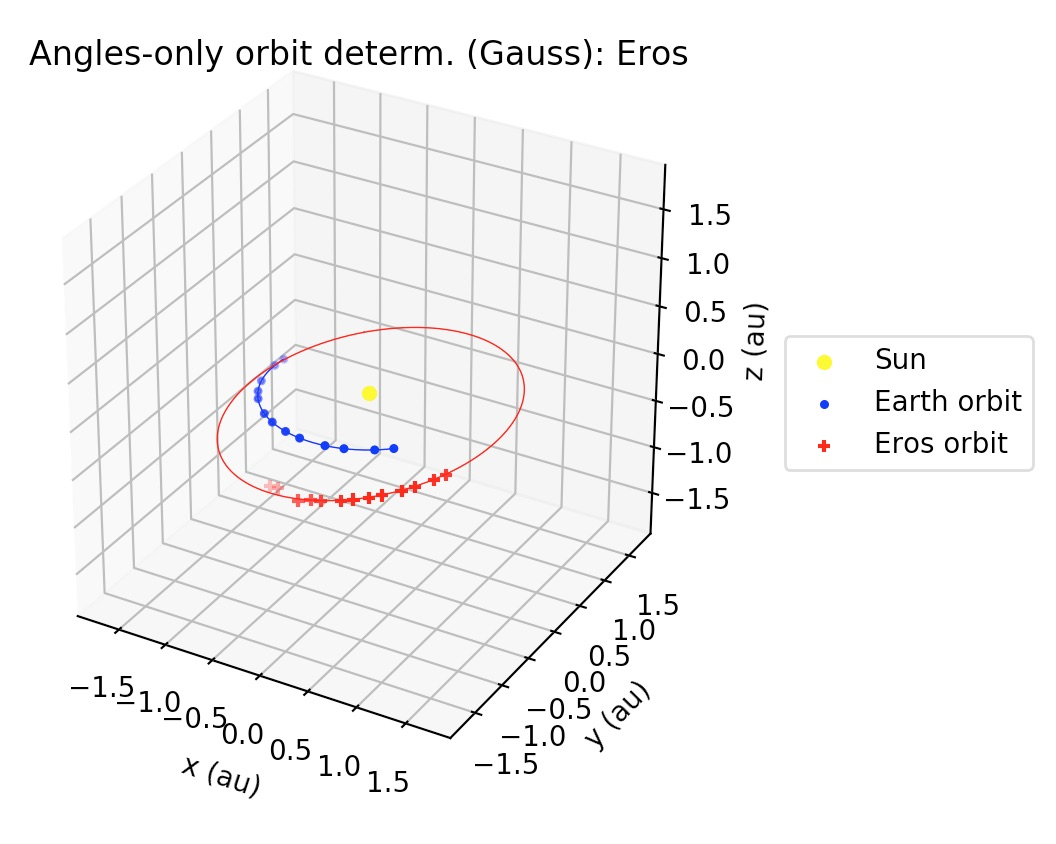## 4. Least-squares

The least-squares functionality developed during my GSoC project may be thought of as differential corrections to the orbits computed by the Gauss method. That is, once a preliminary orbit has been determined by the Gauss method, then the best-fit of the observations to a Keplerian orbit in terms of the observed minus computed residuals (the so-called O-C residuals) may be found by a least-squares procedure. It is worthwhile to mention that in the current implementation all the observations have the same weight in the construction of the O-C residuals vector.

### 4.1 Earth-orbiting bodies: `gauss_LS_sat`

Similar to `gauss_method_sat` in call signature, but after applying the Gauss method to the subset of the observations defined by `obs_arr`, this function applies a least-squares procedure to the whole set of observations (unless otherwise selected by the user using the optional argument `obs_arr_ls`; see documentation), returning the Keplerian elements of the best-fit to the observations. Orbital elements are returned in kilometers, UTC seconds and degrees, and they are referred to the (equatorial) ICRF frame. More details may be found in the documentation and the examples.

Example output: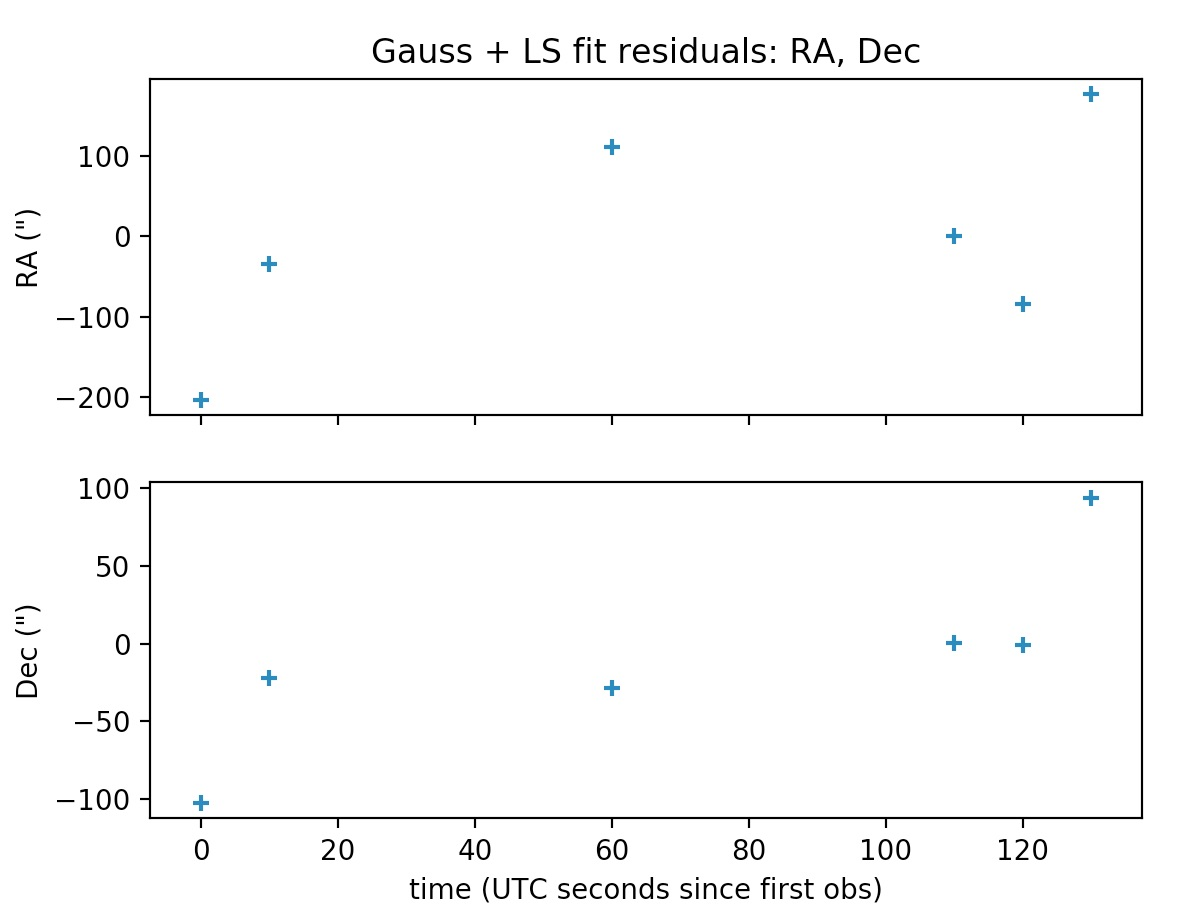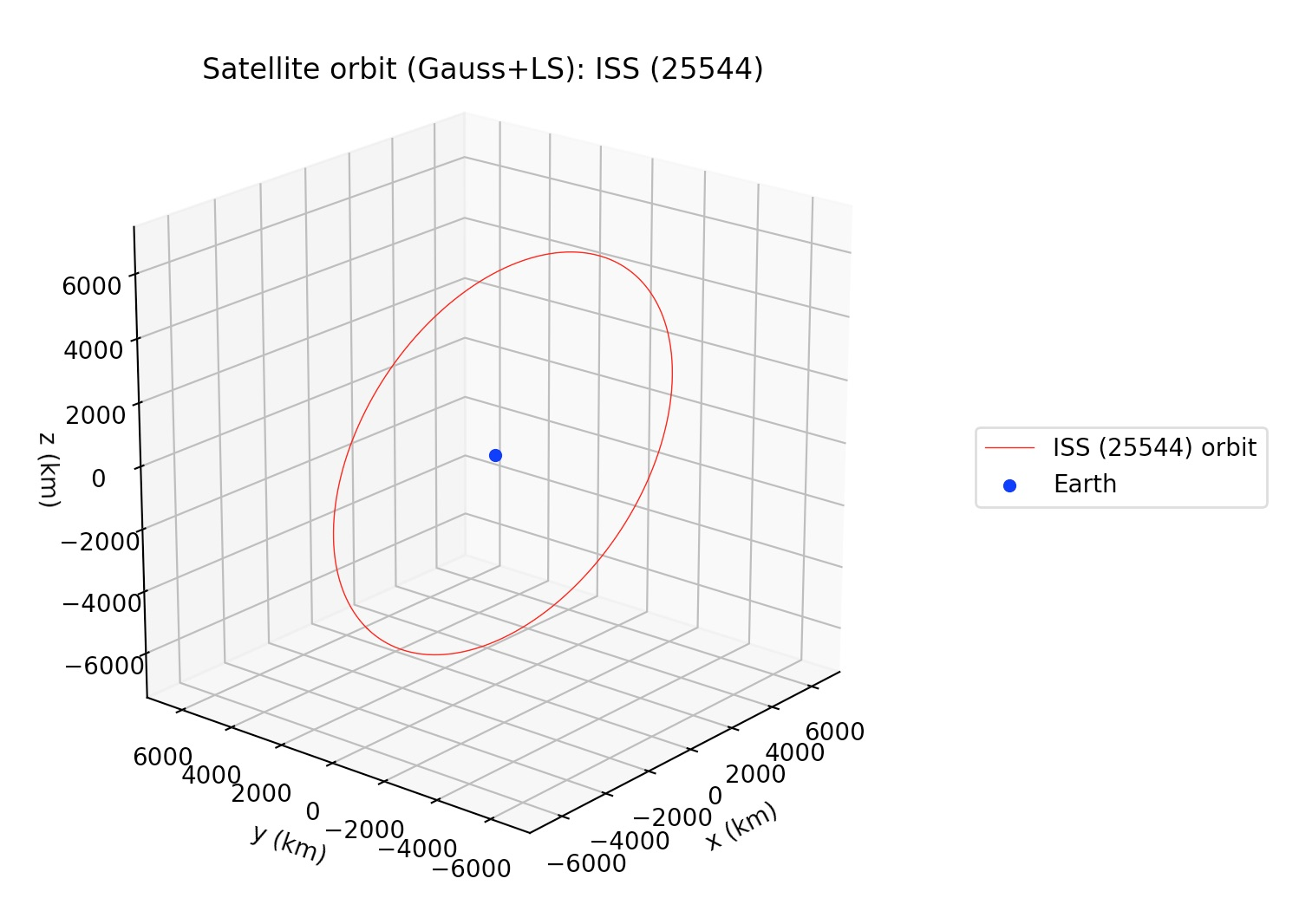### 4.2 Sun-orbiting bodies: `gauss_LS_mpc`

Similar to `gauss_method_mpc` in call signature, but after applying the Gauss method to the subset of the observations defined by `obs_arr`, this function applies a least-squares procedure to the whole set of observations (unless otherwise selected by the user using the optional argument `obs_arr_ls`; see documentation), returning the Keplerian elements of the best-fit to the observations. Orbital elements are returned in astronomical units, TDB days and degrees, and they are referred to the mean J2000.0 ecliptic heliocentric frame. More details may be found at the examples and the documentation.

Example output: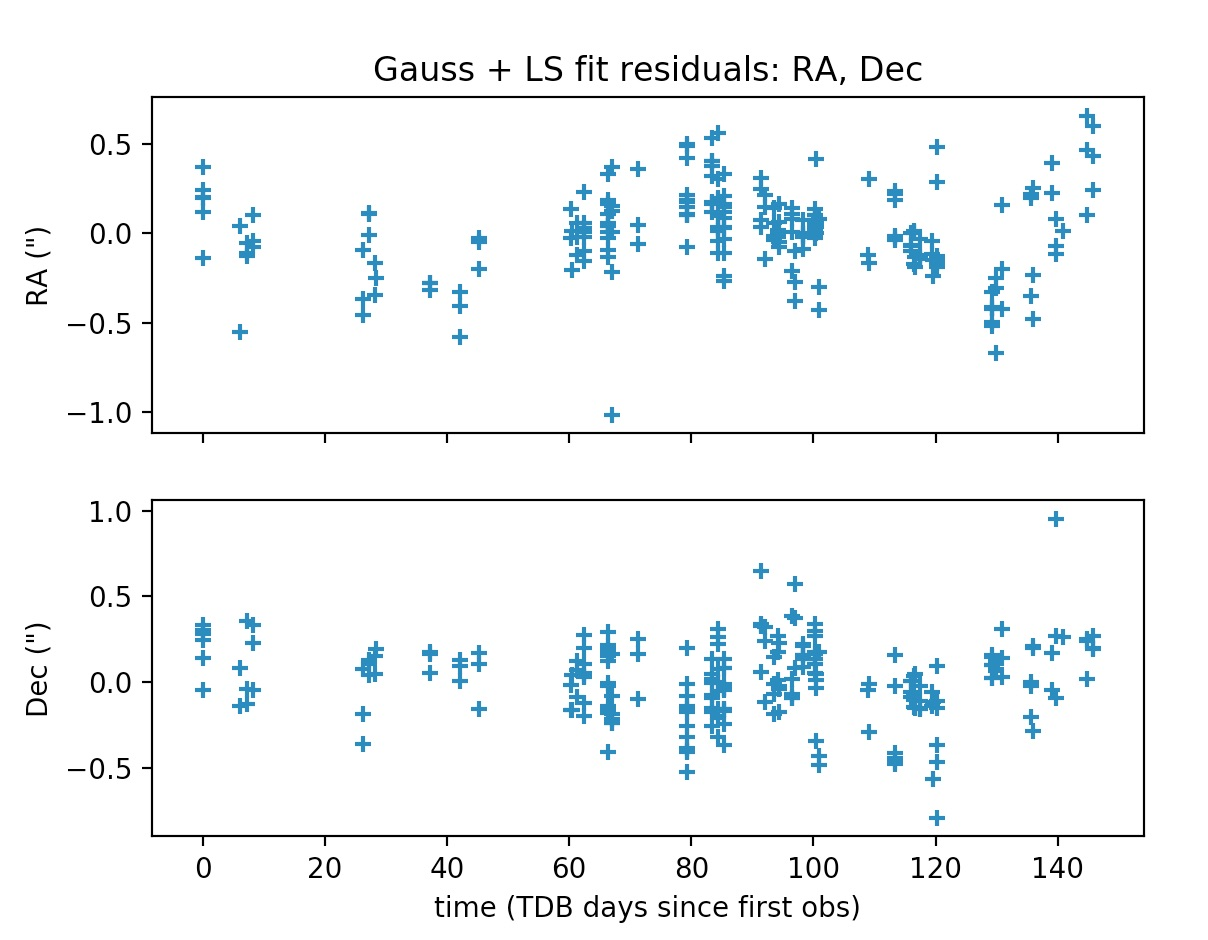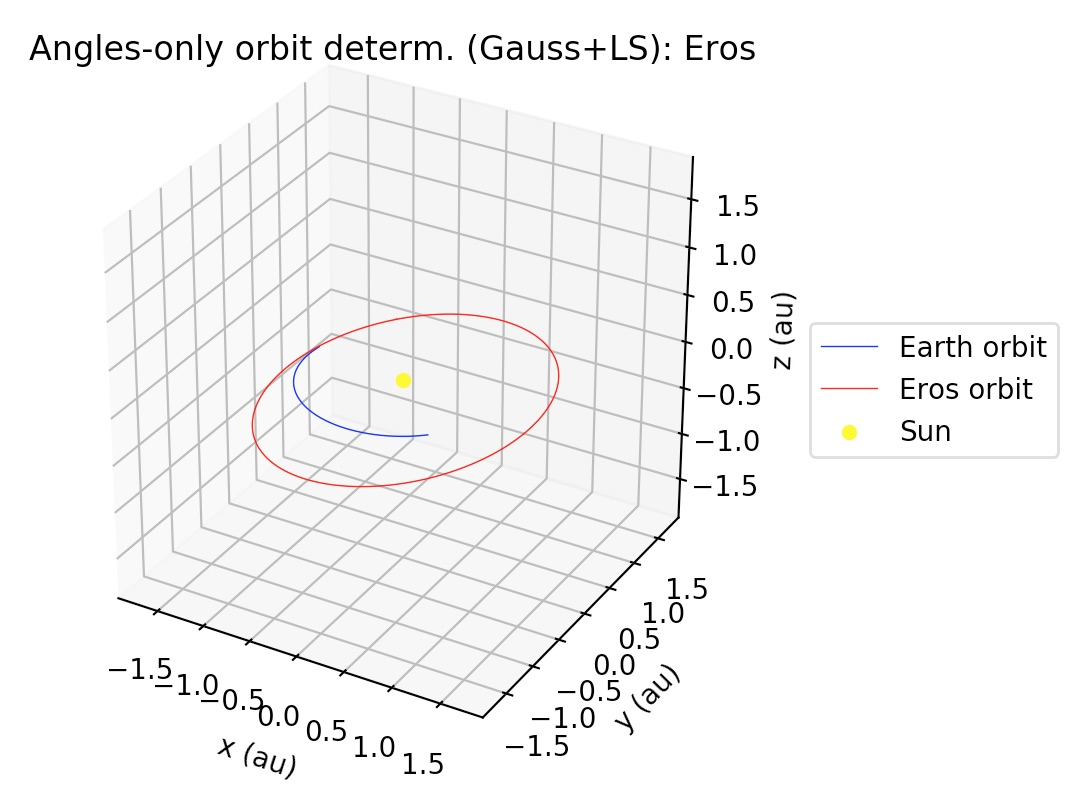## 5. Acknowledgments and credits

We wish to thank the satobs.org community, who gave us valuable comments and suggestions, and also provided us with observations of the ISS and other civilian satellites. We also wish to thank the Mathematical Physics group at the University of Pisa, for all the helpful discussions and feedback which contributed to this work.

## 6. TODOs

• Allow the user to select ephemerides from `astropy`: currently, the user is not allowed to specify the ephemerides to be used in the orbit determination of Sun-orbiting bodies.
• Add perturbations: for Sun-orbiting bodies, add planetary and non-gravitational perturbations; for Earth-orbiting bodies, add oblateness and atmospheric drag to orbit model.
• For IOD-formatted files, allow the use of other „angle subformats“. There are actually 7 ways to codify the angles in IOD format, but currently the code only allows the angle subformat 2. Subformat 2 was chosen because it is the most used in the Satobs community. Some of this subformats involve alt/az measurements, so in a future version the code should know how to handle alt/az measurements in addition to ra/dec measurements. This could be done, e.g., by using the `(alt, az) -> (ra, dec)` conversion functions from the `astropy` library.
• Implement orbit determination algorithms for SDP/SGP4 models from ra/dec measurements.
• Let the user choose between two possible values for the signed difference between two angles, instead of always choosing the shortest signed difference by default.
• Add support for new or non-listed observatories in the MPC or the COSPAR lists of registered observatories.
• Take into account the finite light propagation time, as well as atmospheric refraction.